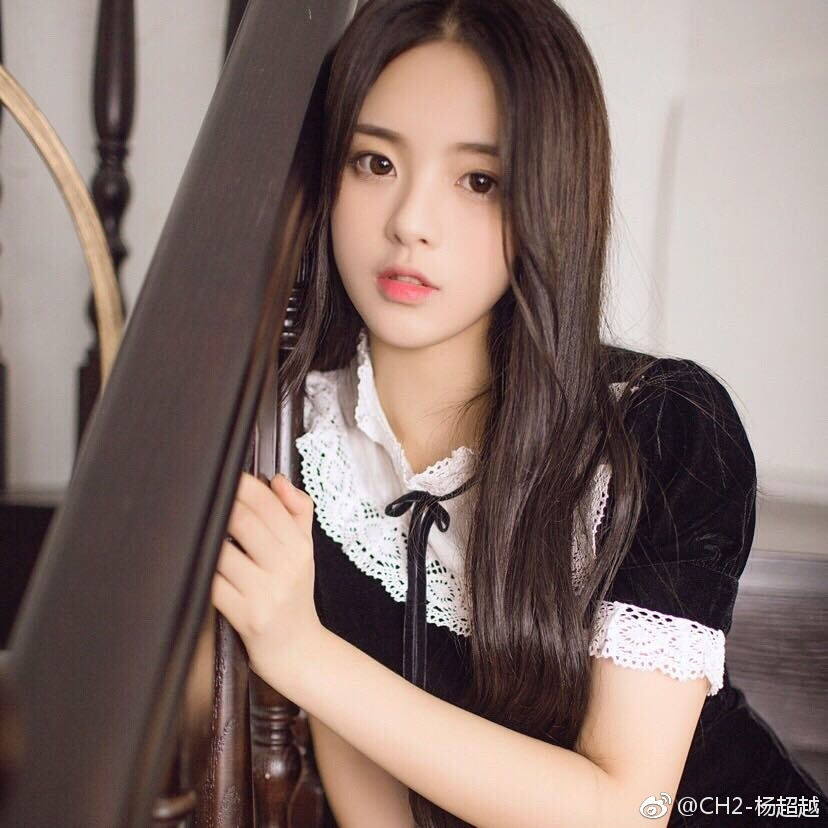# Ascii art from image to characters### Background

I just got interested in Ascii art, and so I searched on the Internet and came up with following code in doing it with Python.

### Code

from PIL import Image, ImageDraw, ImageFont
import operator, bisect

def getChar(val):
"""
return a char for a given gray value
"""
index = bisect.bisect_left(scores, val)  # find index of val in scores

# check and choose the nearer one between current index and former index
if index > 0 and sorted_weights[index] + sorted_weights[index -
1] > 2 * val:
index -= 1
return sorted_weights[index]

def transform(image_file):
"""
return a string containing characters representing each pixel
"""
image_file = image_file.convert("L")  # transform image to black-white
codePic = ''
for h in range(image_file.size):
for w in range(image_file.size):
gray = image_file.getpixel((w, h))
codePic += getChar(maximum * (1 - gray / 255))  # append characters
codePic += '\r\n'  # change lines

return codePic

outputTextFile = 'ycy_ascii.txt'
outputImageFile = 'ycy_ascii.jpg'
fnt = ImageFont.truetype('Courier New.ttf', 10)
chrx, chry = fnt.getsize(chr(32))
normalization = chrx * chry * 255

weights = {}
# get gray density for characters in range [32, 126]
for i in range(32, 127):
sizex, sizey = chrImage.size
ctr = sum(
chrImage.getpixel((x, y)) for y in range(sizey) for x in range(sizex))
weights[chr(i)] = ctr / normalization

weights[chr(32)] = 0.01  # increase it to make blank space ' ' more available
weights.pop('_', None)  # remove '_' since it is too directional
weights.pop('-', None)  # remove '-' since it is too directional
sorted_weights = sorted(weights.items(), key=operator.itemgetter(1))

scores = [y for (x, y) in sorted_weights]
maximum = scores[-1]

resolution = 0.3  # resolution of result ascii image, the higher the better
sizes = [resolution * i for i in (0.665, 0.3122, 4)]
imagefile = base.resize((int(base.size * sizes),
int(base.size * sizes)))

result = transform(imagefile)

# output to text file
asc_text = open(outputTextFile, 'w')
asc_text.write(result)
asc_text.close()

# output to image file and show it
asc_image = Image.new(
'L', (int(base.size * sizes), int(base.size * sizes)), 255)
d = ImageDraw.Draw(asc_image)
d.text((0, 0), result, font=fnt, fill=0)
asc_image.save(outputImageFile)
asc_image.show()
asc_image.close()


### Usage

I run above code in Jupyter Notebook, but it should be easy in adding arguments and changed into .py file.

### Side Notes

1. readinFilePath is the path to the input image file.
2. outputTextFile is the output text file containing ascii characters.
3. outputImageFile is the output image containing ascii characters. I added this because text file always wraps around long lines and results in distorted image.
4. I use font Courier New, you can use other monospace fonts.
5. (0.665, 0.3122, 4) is the ratio between width and height of result image. If using a different font or with a different font size, this should be change a little bit.
7. resolution should be larger than 0, and it can be larger than 1.
8. Yang Chaoyue (杨超越) is so pretty. Congratulations to her becoming a member of “Rocket Girls 101”.

### Input image### Ascii output image### A gif versionWritten on July 6, 2018